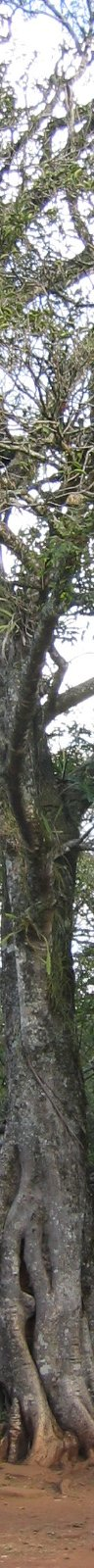#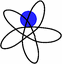BASCOLA: Coloquio de Álgebra en Buenos Aires, Satelital al Coloquio Latinoamericano de Algebra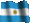Versión en Castellano /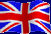English version        Poster

Este encuentro de álgebra se realizará en Buenos Aires entre los días 10 y 12 de agosto de 2005, en el Departamento de Matemática de la Facultad de Ciencias Exactas y Naturales de la UBA.

BASCOLA es un encuentro Satelital al XVI Coloquio Latinoamericano de Álgebra. Sugerimos a los participantes del Coloquio Latinoamericano aprovechar la cercanía geográfica (1 hora en ferry cruzando el Rio de La Plata) para asistir al BASCOLA.

### Comité organizador:

El programa incluirá también un número reducido de comunicaciones.

Auspiciantes: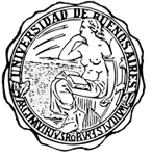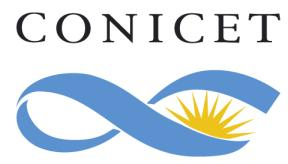Facultad de Ciencias Exactas y Naturales Universidad de Buenos Aires Secyt - PAV 03-120 Secretaría de Ciencia, Tecnología e Innovacion Productiva Consejo Nacional de Investigaciones Científicas y Técnicas www.fcen.uba.ar www.secyt.gov.ar www.conicet.gov.ar

### Títulos y resúmenes de las conferencias

University of Southern Maine, Estados Unidos.

The Geometry of Molecular Evolution: Understanding the Phylogenetic Variety under the general Markov model.

Imagine a collection of currently extant species, assumed to have descended from a common ancestor. A basic problem is to infer a `phylogenetic tree' representing their evolutionary history. Using aligned DNA sequences from the species for data, and some model of the mutation process, this naturally leads to problems in algeraic geometry.

If T is a hypothetical tree, then the general Markov model of sequence evolution requires specifying parameters for the distribution of bases (A, G, T, C) at a root node and a Markov matrix describing the mutation process along each edge of T. This gives a parameterization of an affine variety, and we can use algebraic geometry to understand the closure of the image of this parameterization map, the phylogenetic variety V.

The phylogenetic variety is defined by certain polynomial relationships called `phylogenetic invariants' by biologists or `constraints' by statisticians. In general it is difficult to describe the ideal defining the phylogenetic variety, in part because the number of variables grows exponentially with the number of species.

This talk will discuss ways of finding phylogenetic invariants for the general Markov model. We give an almost complete set-theoretic description of the phylogenetic ideal for the general Markov model for any tree, and show that, in fact, we have an ideal-theoretic description for the special case of two states.

Interestingly, classical constructions such as secant varieties of Segre products appear naturally. In addition, we make connections to related models in statistics and problems in computational complexity theory.

Technion, Israel.

The Brauer group of a field and some of its subgroups. Something old and something new.

The Schur (S(k)) and the projective Schur group (PS(K)) are subgroups of Br(k), the Brauer group of the field k. These groups are defined in terms of certain special central simple algebras that represent the corresponding classes in Br(k). On the other hand it is well known that Br(k) has a cohomological description (Galois cohomology). In the lecture I'll discuss the problem of describing S(k) and PS(k) via cohomology and present some old and new results.

Institut Fourier - Université Grenoble I, Francia.

Spherical varieties and their stable limits

Spherical varieties form a nice class of algebraic varieties where an algebraic group acts with finitely many orbits. Examples include toric varieties, flag varieties, and equivariant compactifications of symmetric spaces. The classification of spherical varieties is an open problem, for which a geometric approach will be presented in the talk. It yields moduli spaces parametrizing spherical varieties together with their equivariant degenerations, stable spherical varieties.

Universidad de Valladolid, Espańa.

Weak equisingular deformations of plane curves

This is join work with Christoph Lossen and Gert-Martin Greuel. Weak equisingular deformations are those which become equisingular in strong sense after some base change. The functor of weak equisingular deformations of a given plane curve singularity becomes relevant in positive characteristic and it has good smoothness and versality properties. Weak deformations also allow to study and compute the equisingular stratum, whose geometry can be described.

University of Massachusetts at Amherst, Estados Unidos.

Binomial Complete Intersections

A binomial ideal in a polynomial ring is an ideal generated by binomials. They are quite ubiquitous in various contexts such as toric geometry, semigroup algebras, and hypergeometric equations. Although binomial ideals are very amenable to Gröbner and standard bases techniques, they also provide some of the "worst-case" examples in computational algebra. Thus, we are interested in algorithms that allow us to obtain information about binomial ideals purely in terms of the data defining them.

In this talk we will discuss how to determine when a binomial ideal is a zero-dimensional complete intersection and, if so, how we may compute the total number of solutions and the total multiplicity of solutions in the coordinate axes. These problems arise naturally in the study of sparse discriminants and in the study of certain systems of differential equations. Joint work with Alicia Dickenstein.

Institut Fourier - Grenoble, Francia.

On General Determinantal Cremona Transformations

Results and survey on related Segre classes, fans and polytopes.

Technische Universität Kaiserslautern, Alemania.

Strong equisingular deformations of curve singularities

I introduce the notion of (strong) equisingular deformations of plane curve singularities in any characteristic and prove the existence, smoothness and algebriicity of the semiumiversal base space.

(Joint work with A. Campillo and C. Lossen)

Université Paris 7, Francia.

From Algebraic K-theory to Hermitian K-theory

We construct a new "characteristic class" between the two theories, which topological analog is the Adams operation. From this characteristic class, we deduce non triviality results in Algebraic K-theory, using Bott periodicity.

Moscow State University

Projective self-dual algebraic varieties and nilpotent orbits

Under different guises dual varieties of projective algebraic varieties have been considered in various branches of mathematics for over a hundred years. In fact, the dual variety is the generalization to algebraic geometry of the Legendre transform in classical mechanics, and the biduality theorem essentially rephrases the duality between the Lagrange and Hamilton--Jacobi approaches in classical mechanics. In 1985-86 L. Ein classified, modulo Hartshorne's famous conjecture, all smooth projective self-dual algebraic varieties. There are only few of them.

In the talk, a method of constructing many singular projective self-dual varieties will be described. They appear as projectivizations of some orbits in Hilbert's null-cones of certain representations of complex semisimple groups. For the adjoint representation, they are precisely distinguished orbits in the sense of Bala and Carter. For the isotropy representations of symmetric spaces, projective self-duality also leads to an equivalent algebraic notion, so-called (-1)-disinguished elements. Kostant-Sekiguchi correpondence then leads to another equivalent algebraic notion, so called compact nilpotent elements of real semisimple algebras. All such orbits admit a complete classification.

Universidad Nacional del Sur, Argentina.

Laura algebras

For an artin algebra A, the left and the right part of the module category have been defined by Happel, Reiten and Smalo, and have been used successfully to study the representation theory of quasi-tilted algebras, shod, weakly shod and laura algebras.

By definition, an indecomposable module M is in the left (right) part of the module category if all its predecessors (successors, respectively) have projective dimension at most one.

In a joint work with I. Assem and M. Lanzilotta we show that it is enough to consider "immediate" predecessors (successors, respectively).

Finally, using the skew group algebras theory, we exhibit several families of weakly shod and laura algebras.

Bates College, Estados Unidos.

The geometry of molecular evolution: new models and varieties

Many models of the evolution of DNA sequences along evolutionary trees lead to parameterizations of algebraic varieties. Understanding these varieties better may well lead to improved methods of inferring from DNA data both evolutionary trees and hidden features of the evolutionary process. After a brief introduction on the basic models, this talk will point toward areas of future development.

Much progress has been made on determining defining equations (= phylogenetic invariants) of varieties for the general Markov (GM) model, and those of its submodels that are `group-based.' However, biologists are very interested in models that capture certain features of evolution that are not present in these models.

For instance, different sites in DNA sequences are widely believed to evolve at different rates. The simplest model of this is one where certain unknown sites are held invariable (I), while others mutate as described by a model such as GM. In geometric terms, this GM+I mixture model leads to a join of a GM variety and a linear space. Understanding the defining equations of the join leads to formulas for recovering the frequencies of invariable sites from data.

In another direction, models with fewer parameters than the GM one, but less rigid structure than the group-based ones, would provide an improved description of biological reality. (Fewer parameters means we can impose more commonality on the evolutionary process along different edges of the tree.) For instance, current models with fewer parameters all assume stable frequencies of the bases A,C,G,T during the mutation process, even though this is known to be violated for many data sets. Developing general stable base distribution models and understanding their associated varieties is a necessary first step even for understanding when the model does not apply. Some phylogenetic invariants for these models can be constructed using hyperdeterminants, but much remains unknown.

This talk will illustrate how algebraic methods arise naturally in the study of molecular evolution, how phylogenetic invariants can be found, and how invariants might play a role in practical applications. Open questions in both theory and application will be mentioned.

Texas A&M University, Estados Unidos.

Syzygies and Toric Varieties

This talk will discuss the interplay between syzygies and toric geometry. First, we'll see that a simple application of Green's theorem gives good bounds on the syzygies of a toric surface. Then I'll discuss joint work with M. Hering and G. Smith on applications of multigraded regularity to syzygies of higher dimensional torics.

Louisiana State University Baton Rouge, Estados Unidos.

On the K-theory of singular varieties

We will present recent work with Cortińas, Haesemeyer and Weibel on the K-theory of singular varieties. In particular we will talk about a solution (in characteristic 0) of a conjecture of Weibel on the vanishing of certain negative K-groups of singular varieties.

Instituto Balseiro, Centro Atómico Bariloche, 8400 Bariloche, Argentina.

ADE rational conformal field theories, face models , paths over graphs and weak Hopf algebras

Rational conformal field theories (RCFT) of sl(2) type are in 1-1 correspondence with ADE graphs. There exists an algebraic structure called the double triangle algebra (DTA) that can be built solely fron the knowledge of the corresponding ADE graph. The tensor product structure of the DTA irreps can be encoded in a graph, called Ocneanu graph of quantum symmetries. Knowledge of this graph enables the calculation of physical quantities characterizing the RCFT in question. Furthermore the DTA are weak Hopf algebras and its elements can be also obtained as the symmetry operators associated to statistical face models built from the ADE graphs and whose critical behaviour is described by the RCFT. A description of these relations will be given.

Northwestern University, Estados Unidos.

Some questions and conjectures about index theorems for the determinant of the cohomology.

After a review of the applications of noncommutative geometry to various index theorems, we formulate some questions about how to extend these results to compute the determinant of the cohomology of an elliptic complex.

Universidad Autónoma de Madrid, Espańa.

On flattening of modules and morphisms

We first discuss the notion of flatenning of modules of finite type, and then we relate it to flatenning of projective morphisms.

We finally apply it to blow ups of singularities over perfect fields.

UC Berkeley, Estados Unidos. Differential operators on zero-dimensional schemes

For a zero-dimensional scheme the filtered structure of its algebra of differential operators and the associated Poisson algebra present a considerable interest.

To determine them is an interesting task already for the case of the infinitesimal neighborhood of order m, of a regular point on an n-dimensional scheme. I will describe how solving that problem shed an unexpected light on the structure and representation theory of finite-dimensional semisimple Lie algebras, including exceptional Lie algebras E6 and E7.

Comentarios mfarinat@dm.uba.ar.

Ultima modificación 14 de julio de 2005.